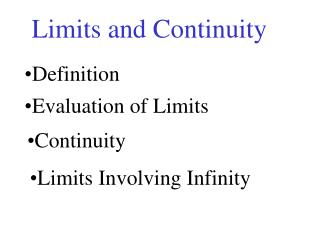DownloadDownload PresentationLimits and Continuity

# Limits and Continuity

Download Presentation## Limits and Continuity

- - - - - - - - - - - - - - - - - - - - - - - - - - - E N D - - - - - - - - - - - - - - - - - - - - - - - - - - -
##### Presentation Transcript

1. Limits and Continuity • Definition • Evaluation of Limits • Continuity • Limits Involving Infinity

2. Limit L a

3. Limits, Graphs, and Calculators Graph 1 Graph 2

4. Graph 3

5. c) Find 6 Note: f (-2) = 1 is not involved • 2

7. Examples What do we do with the x?

8. 1/2 1 3/2

9. One-Sided Limits One-Sided Limit The right-hand limit of f (x), as x approaches a, equals L written: if we can make the value f (x) arbitrarily close to L by taking x to be sufficiently close to the right of a. L a

10. The left-hand limit of f (x), as x approaches a, equals M written: if we can make the value f (x) arbitrarily close to L by taking x to be sufficiently close to the left of a. M a

11. Examples of One-Sided Limit Examples 1. Given Find Find

12. More Examples Find the limits:

13. A Theorem This theorem is used to show a limit does not exist. For the function But

14. Limit Theorems

15. Examples Using Limit Rule Ex. Ex.

16. More Examples

17. Indeterminate Forms Indeterminate forms occur when substitution in the limit results in 0/0. In such cases either factor or rationalize the expressions. Notice form Ex. Factor and cancel common factors

18. More Examples

19. The Squeezing Theorem See Graph

20. Continuity A function f is continuous at the point x = a if the following are true: f(a) a

21. A function f is continuous at the point x = a if the following are true: f(a) a

22. At which value(s) of x is the given function discontinuous? Examples Continuous everywhere Continuous everywhere except at

23. and and Thus F is not cont. at Thus h is not cont. at x=1. F is continuous everywhere else h is continuous everywhere else

24. Continuous Functions If f and g are continuous at x = a, then A polynomial functiony = P(x) is continuous at every point x. A rational function is continuous at every point x in its domain.

25. Intermediate Value Theorem If f is a continuous function on a closed interval [a, b] and L is any number between f (a) and f (b), then there is at least one number c in [a, b] such that f(c) = L. f (b) f (c) = L f (a) a c b

26. Example f (x) is continuous (polynomial) and since f (1) < 0 and f (2) > 0, by the Intermediate Value Theorem there exists a c on [1, 2] such that f (c) = 0.

27. Limits at Infinity For all n > 0, provided that is defined. Divide by Ex.

28. More Examples

29. Infinite Limits For all n > 0, More Graphs

30. Examples Find the limits

31. Limit and Trig Functions From the graph of trigs functions we conclude that they are continuous everywhere

32. Tangent and Secant Tangent and secant are continuous everywhere in their domain, which is the set of all real numbers

33. Examples

34. Limit and Exponential Functions The above graph confirm that exponential functions are continuous everywhere.

35. Asymptotes

36. Examples Find the asymptotes of the graphs of the functions